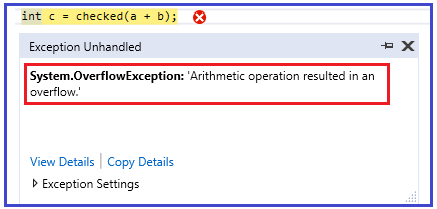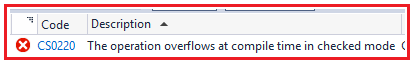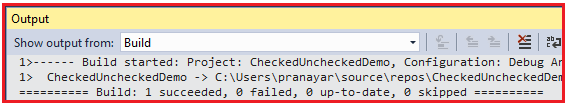# Checked and unchecked keyword in C#

## Checked and unchecked keyword in C#

In this article, I am going to discuss the need and use of Checked and unchecked keyword in C# with examples. Please read our previous article, where we discussed the difference between Convert.ToString and ToString method in C#. The C# provides checked and unchecked keyword which are used to handle integral type exceptions.

##### Why do we need Checked and unchecked keyword in C#?

According to MSDN, The checked keyword in C# is used to explicitly enable overflow checking for integral-type arithmetic operations and conversions.

The unchecked keyword in C# is used to suppress overflow-checking for integral-type arithmetic operations and conversions.

Here, overflow checking means when the value of any integral-type exceeds its range, it does not raises any exception, instead it will give us unexpected or garbage results. If this is not clear at the moment, then don’t worry we will try to understand the above two points with examples.

##### Example:

First, create a console application. Now, for demonstration purpose let’s take “int” data type and see what the maximum value it can hold. To do so, please modify the Program class as shown below.

```using System;
namespace CheckedUncheckedDemo
{
class Program
{
static void Main(string[] args)
{
Console.WriteLine(int.MaxValue);
}
}
}```

Output: 2147483647

##### Example without Checked Keyword:

Now, let’s see where the checked keyword can help us in making your code more useful. In the following example, you can see that we have three integer variables. The integer variables a and b holds the maximum value that an integer can hold. Then we just simply add the integer a and b and stored it in the third integer variable i.e. c.

```using System;
namespace CheckedUncheckedDemo
{
class Program
{
static void Main(string[] args)
{
int a = 2147483647;
int b= 2147483647;

int c = a + b;

Console.WriteLine(c);
}
}
}```

Now run the application and see the output.

Output: -2

As you can see it display -2, but this is we were not expecting. What we except is some error (overflow) or exception. This is where the Checked keyword helps us to achieve and throws overflow exception.

##### Example: Using Checked Keyword

The code example uses checked keyword. As we use the checked keyword, it should throws runtime exception rather than displaying -2.

```using System;
namespace CheckedUncheckedDemo
{
class Program
{
static void Main(string[] args)
{
int a = 2147483647;
int b= 2147483647;

int c = checked(a + b);

Console.WriteLine(c);
}
}
}```

Now, when you run the application, you should get the following OverflowException as expected.In simple words, we can say that the checked keyword is used in scenarios where you want to ensure that your left hand side data type is not getting overflow

##### Unchecked keyword in C#:

Let us understand the need and use of unchecked keyword in C#. The unchecked keyword behaves almost the same way as the default behavior of the compiler.

Let’s prove that the above point. So, modify the Program class as shown below and then see the output.

```using System;
namespace CheckedUncheckedDemo
{
class Program
{
static void Main(string[] args)
{
int a = 2147483647;
int b= 2147483647;

int c = unchecked(a + b);

Console.WriteLine(c);
}
}
}```

As shown in the above code, we have have just added the unchecked keyword in front of the arithmetic expression of the c variable. Now, run your application and you should get the following output..

###### Output: -2

So this proves that the unchecked keyword works almost the same way as the default compiler works. Now the question that should comes to your mind is, when the default compiler works same as unchecked keyword then what is the exact use of it.

Now, let’s see a simple example to understand the exact need of unchecked keyword in C#. Please modify the Program class as shown below.

```using System;
namespace CheckedUncheckedDemo
{
class Program
{
static void Main(string[] args)
{
const int a = 2147483647;
const int b= 2147483647;

int c = a + b;

Console.WriteLine(c);
}
}
}```

As you can see in the above code that we have declared variable a and b as const int. now, when you try to compile the project, you should get the following compile time error.If you want to bypass this behavior then you need to use the unchecked keyword in C#. Please modify the Program class as shown below which will help you to achieve this task.

```using System;
namespace CheckedUncheckedDemo
{
class Program
{
static void Main(string[] args)
{
const int a = 2147483647;
const int b= 2147483647;

int c = unchecked(a + b);

Console.WriteLine(c);